# Common Core: High School - Geometry : Congruence

## Example Questions

1 2 7 8 9 10 11 12 13 15 Next →

### Example Question #1 : Construct Inscribed Figures (Equilateral Triangles, Squares, Regular Hexagons): Ccss.Math.Content.Hsg Co.D.13

Determine whether the statement is true or false:

For an object to be inscribed in a circle only one of its points must lie within the circle.

True

False

False

Explanation:

To determine whether this particular statement is true or false, first understand what the term "inscribed" means.

"Inscribed" means to draw inside of. Therefore, for any object to be inscribed in a circle that means all points of that object must also be inside the circle.

Thus the statement, "For an object to be inscribed in a circle only one of its points must lie within the circle." is false.

### Example Question #2 : Construct Inscribed Figures (Equilateral Triangles, Squares, Regular Hexagons): Ccss.Math.Content.Hsg Co.D.13

Determine whether the statement is true or false:

Polygons can be both inscribed inside a circle and have circles inscribed inside of them.

True

False

True

Explanation:

To determine whether this particular statement is true or false, first understand what the term "inscribed" means.

"Inscribed" means to draw inside of. Therefore, any and all polygons can be inscribed in a circle and circles can be inscribed in all polygons.

Thus, the statement, "Polygons can be both inscribed inside a circle and have circles inscribed inside of them." is true.

### Example Question #141 : Congruence

Which of the following images is a diamond that is inscribed in a circle?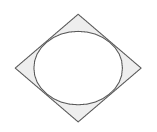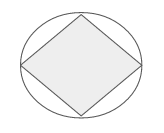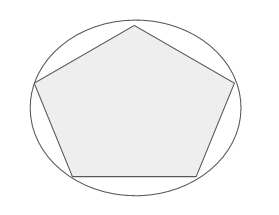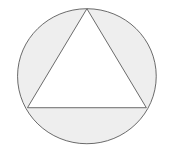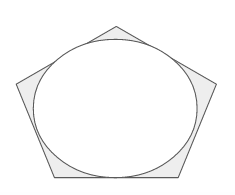Explanation:

To determine which image illustrates a diamond that is inscribed in a circle, first understand what the term "inscribed" means.

"Inscribed" means to draw inside of. Therefore, a diamond inscribed in a circle means the diamond will be drawn inside of a circle.

The image that illustrates this is as followed.### Example Question #142 : Congruence

Which of the following images is a circle that is inscribed in a diamond?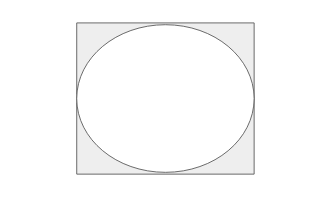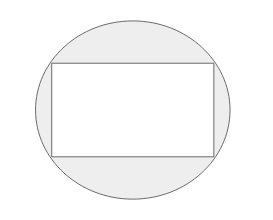Explanation:

o determine which image illustrates a circle that is inscribed in a diamond, first understand what the term "inscribed" means.

"Inscribed" means to draw inside of. Therefore, a circle inscribed in a diamond means the circle will be drawn inside of a diamond.

The image that illustrates this is as followed.1 2 7 8 9 10 11 12 13 15 Next →

### All Common Core: High School - Geometry Resources